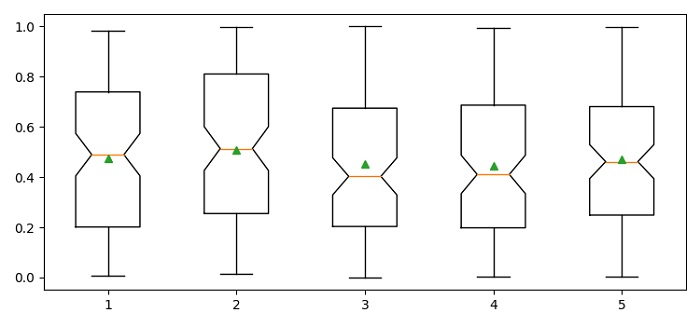# How to show mean in a box plot in Python Matploblib?

MatplotlibPythonData Visualization

To show mean in a box plot, we can use showmeans=True in the argument of boxplot() method.

## Steps

• Set the figure size and adjust the padding between and around the subplots.

• Create a random dataset.

• Create a new figure or activate an existing figure using figure() method.

• Add an axes to the current figure as a subplot arrangement.

• Make a box and whisker plot using boxplot() method.

• To display the figure, use show() method.

## Example

import matplotlib.pyplot as plt
import numpy as np

plt.rcParams["figure.figsize"] = [7.50, 3.50]
plt.rcParams["figure.autolayout"] = True
data = np.random.rand(100, 5)
fig = plt.figure()
plt.show()